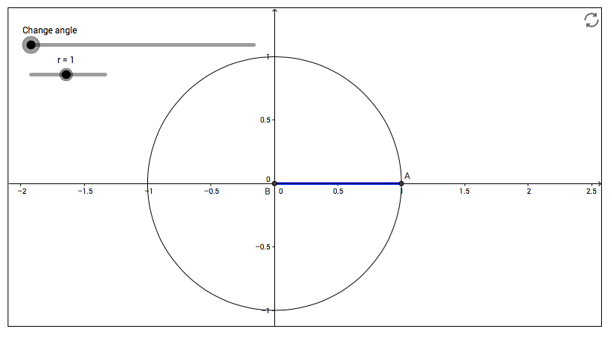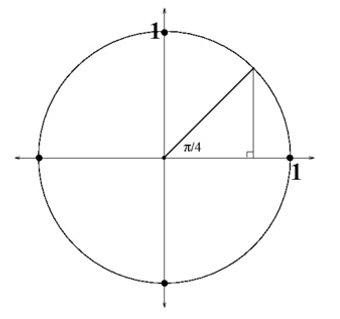# Unit Circle and Trigonometric Functions

## Objective

Convert between degrees and radians and evaluate trigonometric functions written in radians.

## Common Core Standards

### Core Standards

?

• F.TF.A.1 — Understand radian measure of an angle as the length of the arc on the unit circle subtended by the angle.

• F.TF.A.3 — Use special triangles to determine geometrically the values of sine, cosine, tangent for π/3, π/4 and π/6, and use the unit circle to express the values of sine, cosine, and tangent for π-x, π+x, and 2π-x in terms of their values for x, where x is any real number.

?

• G.C.B.5

## Criteria for Success

?

1. Describe the reasoning behind a “radian.”
2. Identify the fraction of a circle that is represented by each reference angle and apply that thinking to radians.
4. Identify sine, cosine, tangent, and the quadrant for any number of radians provided.

## Tips for Teachers

?

• Make sure students know how to switch between degrees and radians using their calculators—it will be helpful to mix up problems in the problem set to require students to pay attention to the units.

## Anchor Problems

?

### Problem 1

If ${{{{{360}}}}}$ degrees is ${2{\pi}}$ radians, how many degrees are ${\pi}$ radians?
If ${{{{{360}}}}}$ degrees is ${2{\pi}}$ radians, how many degrees are $\frac{{\pi}}{3}$ radians?
If ${{{{{360}}}}}$ degrees is ${2{\pi}}$ radians, how many radians are ${90}$ degrees?
If ${{{{{360}}}}}$ degrees is ${2{\pi}}$ radians, how many radians are ${540}$ degrees?
If ${{{{{360}}}}}$ degrees is ${2{\pi}}$ radians, how many degrees are $-\frac{5{\pi}}{4}$ radians?

### Problem 2

How many radii does it take to go around the whole circle? How would you represent this in terms of pi?#### References

What is a Radian? by Sam Shah is made available by GeoGebra under the CC BY-NC-SA 3.0 license. Copyright © International GeoGebra Institute, 2013. Accessed Feb. 26, 2018, 1:22 p.m..

### Problem 3

Use the unit circle and indicated triangle below to find the exact value of the sine and cosine of the special angle ${\frac{\pi}{4}}$.#### References

Illustrative Mathematics Special Triangles 1

Special Triangles 1, accessed on Feb. 26, 2018, 1:26 p.m., is licensed by Illustrative Mathematics under either the CC BY 4.0 or CC BY-NC-SA 4.0. For further information, contact Illustrative Mathematics.

## Problem Set

?

The following resources include problems and activities aligned to the objective of the lesson that can be used to create your own problem set.

• Include straightforward practice converting between radians and degrees.

?

### Problem 1

Convert ${60^\circ}$ to radians.

#### References

EngageNY Mathematics Algebra II > Module 2 > Topic A > Lesson 9Exit Ticket, Question #1

Algebra II > Module 2 > Topic A > Lesson 9 of the New York State Common Core Mathematics Curriculum from EngageNY and Great Minds. © 2015 Great Minds. Licensed by EngageNY of the New York State Education Department under the CC BY-NC-SA 3.0 US license. Accessed Dec. 2, 2016, 5:15 p.m..

### Problem 2

Convert ${-\frac{\pi}{2}}$ rad to degrees.

#### References

EngageNY Mathematics Algebra II > Module 2 > Topic A > Lesson 9Exit Ticket, Question #2

Algebra II > Module 2 > Topic A > Lesson 9 of the New York State Common Core Mathematics Curriculum from EngageNY and Great Minds. © 2015 Great Minds. Licensed by EngageNY of the New York State Education Department under the CC BY-NC-SA 3.0 US license. Accessed Dec. 2, 2016, 5:15 p.m..

### Problem 3

Evaluate ${\mathrm{cos}\frac{3\pi}{4}}$.

### Problem 4

If you evaluated ${\mathrm{sin}3.1}$ on your calculator in radian mode, would the answer be positive or negative? Why?